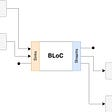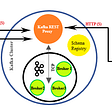# Solving Recursion Coding Problems

## Solving Recursion Coding Problems with Explanations

As tree is a recursive data structure, many tree related problems can be solved recursively, please find these problem from binary tree and binary search tree.

# 1. Question list

1. Climbing stairs G4G Leetcode `Easy`
2. Longest univalue path Leetcode `Easy` `Binary Tree`
3. Minimum Distance Between BST Nodes Leetcode `Easy` `BST`
4. Reverse a linked list using recursion `Easy`
5. Reverse a string using recursion Techie `Easy` todo
6. Number of paths G4G Leetcode `Medium` todo

# 2. C++ Implementation

## Climbing stairs

You are climbing a staircase. It takes n steps to reach the top. Each time you can either climb 1 or 2 steps. In how many distinct ways can you climb to the top?

Method 1: Recursion

`int climbStairs(int s) {     if (n <= 2)//0,1,2,3,5,8        return n;    return climbStairs(n-1) + climbStairs(n-2); }`

Explanation

1. As each time you can either climb 1 or 2 steps, the person can reach the n’th stair from either (n-1)’th stair or from (n-2)’th stair, so we can add up ways of (n-1) and (n-2) to get the result for n stairs.
2. Note that it is slightly different from than Fibonacci numbers shown below. Thus we have to define the base case if n ≤ 2.
3. Time complexity is exponential. T(n) = T(n-1) + T(n-2) + 1 = 2^n = O(2^n), space complexity: O(n). Since climbStairs(n-1) gets evaluated completely before climbStairs(n-2), there will never be more than n levels of recursion.
`Fibonacci numbers:Pattern 1: 0,1,1,2,3,5,8...Pattern 2: 1,1,2,3,5,8,13...Our numbers:0,1,2,3,5,8,13...`

Method 2: Dynamic programming-memoization, ref: G4G.

`int climbStairs(int n) {    unordered_map<int, int>steps;    steps = 0;    steps = 1;    steps = 2;    for (int i = 3; i <= n; i++){        steps[i] = steps[i-1] + steps[i-2];    }    return steps[n];}`

Explanation

1. Use Bottom-Up Approach: Suppose we need to solve the problem for N, We start solving the problem with the smallest possible inputs and store it for the future. Now as you calculate for the bigger values use the stored solutions (solution for smaller problems).
2. Time Complexity: O(n) , Space Complexity : O(n)

We can also use Top-Down Approach to solve this problem which still uses recursion but instead of calculating each sub problem, first check if solution is already available, if yes then use it else calculate and store it for future. Ref: https://algorithms.tutorialhorizon.com/introduction-to-dynamic-programming-fibonacci-series/

Method 3: Improved dynamic programming

`int climbStairs(int n) {    int result = n; //if n < 3, return n (0, 1, 2)    int a = 1, b = 2;    for (int i = 3; i <= n; i++){        result = a + b;        a = b;        b = result;    }    return result;}`

Explanation

1. We can optimize the space used in method 2 by storing the previous two numbers only because that is all we need to get the next number in the series.
2. Time Complexity: O(n), Extra Space: O(1).

## Longest univalue path

Given a binary tree, find the length of the longest path where each node in the path has the same value. This path may or may not pass through the root.

`int postOrder(TreeNode* t, int &result) {    if(t == NULL) return 0;    int max_l = postOrder(t->left, result);    int max_r = postOrder(t->right, result);    //extend 1 or to be 0.     max_l = (t->left && t->left->val == t->val) ? ++max_l:0;    max_r = (t->right && t->right->val == t->val) ? ++max_r:0;        result = max(result, max_l + max_r); //update the final result        //return to the parent’s parent node    //only through one side is valid path    return max(max_l, max_r); }int longestUnivaluePath(TreeNode* root) {    int result = 0;    postOrder(root, result);    return result;}`

Explanation

1. We use a helper to complete as the root node may not be included which means we can’t return the longest path so far for each recursive call. Instead, we need to track the longest path for each step and return only the greater one among left and right paths.
2. The reason we either add 1 to max_l or max_r or set it to be 0 is that: if the value of the root node is different than that of (say left child), the number of edges to the left child will be 0.
3. Time Complexity: O(N), where N is the number of nodes in the tree. We process every node once.
4. Space Complexity: O(H), where H is the height of the tree. Our recursive call stack could be up to H.
5. The reason we can’t return the longest path so far for each recursive call is illustrated below. If we return the longest path so far, we will get the result of 3 instead of the correct answer 2. Because only through one side is a valid path. The correct way to handle it is to update the final result (twice in the figure below).

The moments where I got stuck

I have tried several ways to implement using only one function but ended up in failure. The one pasts the most test cases is shown below. but it fails in the case when the tree looks like above.

`int longestUnivaluePath(TreeNode *root){    if (!root)        return 0;    int left = longestUnivaluePath(root->left);    int right = longestUnivaluePath(root->right);    int max = left > right ? left: right;    if (left >= right && root->left && root->val == root->left->val)        max++;    if (left <= right && root->right && root->val == root->right->val)        max++;    if (left!=right && root->left && root->right && root->val == root->right->val && root->val == root->left->val)        max++;    return max;}`

For this kind of question, I concluded that before figuring out a solution, it’s better to understand some test cases and the expected answers. If we first draw several binary trees with different patterns, it may be easier to notice we may need a helper function. Don’t start coding until we have a concrete solution.

## Minimum Distance Between BST Nodes

Given a Binary Search Tree (BST) with the root node `root`, return the minimum difference between the values of any two different nodes in the tree.

`void helper(TreeNode* root, int &res, int &pre){    if(root == nullptr) return;    helper(root->left, res, pre);    if(pre != -1) res = min(res, root->val - pre);    pre = root->val;    helper(root->right, res, pre);}int minDiffInBST(TreeNode* root) {    int res = INT_MAX, pre = -1;    helper(root, res, pre);    return res;}`

Explanation

`          4        /   \      2      6     / \        1   3helper(4, INT_MAX, -1)  helper(2, INT_MAX, -1)    helper(1, INT_MAX, -1)      helper(null, INT_MAX, -1) //1’s left    pre = 1      helper(null, INT_MAX, 1) //1’s right  res = min(INT_MAX, 2-1) = 1  pre = 2    helper(3, 1, 2)      helper(null, 1, 2) //3’s left    res = min(1, 3-2) = 1    pre = 3      helper(null, 1, 3) //3’s right, end of 4’s left treeres = min(1, 4-3) = 1pre = 4  helper(6, 1, 4) //start of 4’s right tree    helper(null, 1, 4) //6’s left  pre = 6    helper(null, 1, 6) //6’s right  res = min(1, 6-4) = 1 //end of 4’s right`
1. We can use in-order traversal to compare the current node’s value and previous value and update the result if we found a smaller one
2. T = O(n): number of nodes = number of recursion call (ignoring the null), M = O(logn): depth of tree

The moments where I got stuck

1. I thought we only need to compare the value of the current node and its children and ignored that we need to compare the value of the current node with the largest node (rightmost children) of its left tree and smallest node (left most children) of its right tree (e.g. 5–4 = 1 below), we can achieve this by inorder traversal.
`          5        /   \      2      8     / \        0   4`

2. Draw the recursion calling process to help analyze the problem.

## Reverse a linked list using recursion

`ListNode* reverse(ListNode* head) {    if(head == nullptr || head->next == nullptr) return head;    ListNode *node = reverse(head->next);    head->next->next = head;    head->next = nullptr;    return node;}`

Explanation: article, video

1. The key is to return the last element as a new head and keep returning this new head in each recursive call, so we will eventually get the new head after all recursive is called.
`1->2->3->4->nullreverse(1)  reverse(2)    reverse(3)      reverse(4), 4->next is null, return 4 as head, list: 4    node=4    3->next->next = 3 (4->next=3), list: 4->3    3->next = nullptr, list:4->3->null    return node (4)  node=4  2->next->next = 2(3->next=2), list:4->3->2  2->next = nullptr, list: 4->3->2->null  return node (4)node=41->next->next = 1(2->next=1), list: 4->3->2->11->next = nullptr, list:4->3->2->1->nullreturn node (4)`

The moments where I got stuck

1. I found difficulties in getting the new head after the last call (i.e reverse(1)) is returned and set the last node’s next to null. The code above can solve this problem.

## Reverse a string using recursion

`void reverse(string &s, int l, int h){    if(l < h){        swap(s[l], s[h]);        reverse(s, l+1, h-1);    }}reverse(s, 0, s.lenght()-1);`

For more recursion questions, see Leetcode, G4G, and Techie Delight

--

--

--

## More from Coding Interview Questions in C++

Coding Interview Questions in C++ with question links, c++ code and explanation

## Flutter at OY! Indonesia: Migration Strategy and Architecture## Confluent’s Kafka REST Proxy, The Silk Route for Data Movement to Operational Kafka Cluster## How to Implement RPA in Banking & Finance?## Building and Publishing a Docker Image to Amazon ECR using Bitbucket Pipelines## Black Swans — Rise and Fall of Automation## Understanding and Measuring the OSS Vulnerabilities beyond Code## Web Scraping with Beautiful Soup — Parent and Sibling Elements## Content Management — Designing HTML All The Things## Larry | Peng Yang

Software Engineer in Tokyo. Aim to understand computer science very well. LinkedIn: https://www.linkedin.com/in/peng-larry-yang-9a794561/

## DSA #4 Binary Tree and Binary Search Tree## LeetCode 414. Third Maximum Number## A fun but tricky concept — Recursion